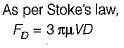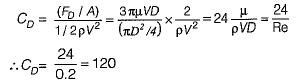Courses

# Test: Forces on Submerged Bodies

## 10 Questions MCQ Test Fluid Mechanics | Test: Forces on Submerged Bodies

Description
This mock test of Test: Forces on Submerged Bodies for Mechanical Engineering helps you for every Mechanical Engineering entrance exam. This contains 10 Multiple Choice Questions for Mechanical Engineering Test: Forces on Submerged Bodies (mcq) to study with solutions a complete question bank. The solved questions answers in this Test: Forces on Submerged Bodies quiz give you a good mix of easy questions and tough questions. Mechanical Engineering students definitely take this Test: Forces on Submerged Bodies exercise for a better result in the exam. You can find other Test: Forces on Submerged Bodies extra questions, long questions & short questions for Mechanical Engineering on EduRev as well by searching above.
QUESTION: 1

Solution:
QUESTION: 2

Solution:
QUESTION: 3

### A body is called a stream-line body when

Solution:
QUESTION: 4

The optimum amount of stream-lining is that for which

Solution:
QUESTION: 5

Pressure drag results from

Solution:
QUESTION: 6

The boundary or profile drag is essentially made up of

Solution:

The total drag (sometimes called as profile drag) of a body is made up of two parts: Frictional drag and pressure drag (or from drag), depending on whether the shear stresses or pressure differences cause it.

QUESTION: 7

In calculating the drag force using CD the area used is

Solution:

Planform area is the area of body as seen from above. This area is used for thin, flat surfaces where frictional forces are predominant as for example: flat plate, flow, airplane wings and hydrofoils.

QUESTION: 8

Stake’s law is valid up to a maximum Reynolds number of

Solution:
QUESTION: 9

A very thin sphere is settling down in a viscous liquid at a Reynolds number of 0.2. Its drag coefficient is

Solution:QUESTION: 10

The Karman vortex trail' occurs

Solution: